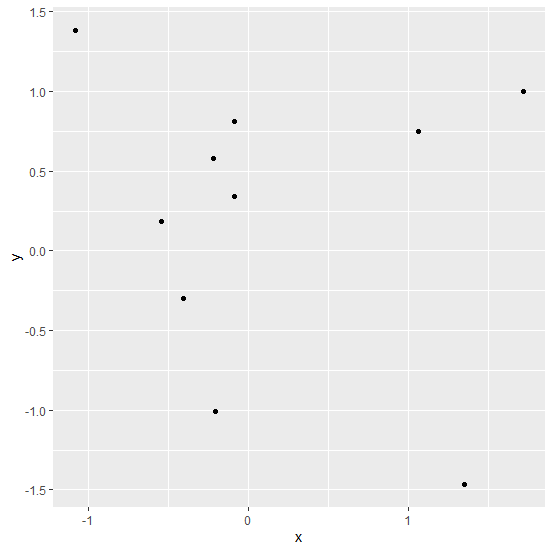# How to detach a package in R?

R ProgrammingServer Side ProgrammingProgramming

To detach a package in R, we can simply use the detach function. But we need to remember that once the package will be detached there is no way to use any of the functions of that particular package. We make this mistake if we forget about detachment. For example, if we detach ggplot2 package using detach function detach(package:ggplot2,unload=TRUE) and again run the ggplot or qplot function then there will be an error.

## Example

Consider the below data frame −

Live Demo

> x<-rnorm(10)
> y<-rnorm(10)
> df<-data.frame(x,y)
> df

## Output

x y
1 -0.09124881 0.8106691
2 -0.20521435 -1.0067072
3 -1.07904498 1.3867400
4 1.34461945 -1.4676405
5 -0.21731862 0.5801624
6 -0.54413731 0.1817524
7 1.05737101 0.7518694
8 -0.08566360 0.3428287
9 -0.40894417 -0.2992289
10 1.71507507 0.9979026

> library(ggplot2)
> ggplot(df,aes(x,y))+geom_point()

## OutputDetaching the ggplot2 package −

> detach(package:ggplot2,unload=TRUE)

Now again creating the plot −

> ggplot(df,aes(x,y))+geom_point()
Error in ggplot(df, aes(x, y)) : could not find function "ggplot"